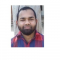## How to display fraction in a plot title using ggplot2 in R?Updated on 23-Nov-2021 06:11:56
To display fraction in a plot title using ggplot2 in R, we can use frac function inside ggtitle function of ggplot2 package. Generally, fraction is displayed as X/Y but frac function helps to create the fraction in over form.Check out the below example to understand how over form of fraction can be displayed in a plot title using ggplot2.ExampleFollowing snippet creates a sample data frame −x

## How to find the sequence of correlation between variables in an R data frame or matrix?Updated on 23-Nov-2021 05:51:00
To find the sequence of correlation between variables in an R data frame or matrix, we can use correlate and stretch function from corrr package.For example, if we have a data frame called df then we can find the sequence of correlation between variables in df by using the below given command −df%>% correlate() %>% stretch() %>% arrange(r)Example 1Following snippet creates a sample data frame −x1% + arrange(r) Correlation method: 'pearson' Missing treated using: 'pairwise.complete.obs' # A tibble: 25 x 3OutputIf you execute all the above given snippets as a single program, it generates the following output −  ... Read More

## How to create a matrix with vectors as elements in R?Updated on 23-Nov-2021 05:45:59
To create a matrix with vectors as elements in R, we can create arrays because an array contains matrices with vectors as elements.Check out the below given examples of arrays and vectors extracted by the arrays to understand how matrices stored in an array represent vectors as elements.Example 1Following snippet creates arrays consisting of matrices −Array

## How to create a vertical line in a time series plot in base R?Updated on 23-Nov-2021 05:42:07
To create a time series plot, we can use simply apply plot function on time series object and if we want to create a vertical line on that plot then abline function will be used with v argument.For example, if we have a time series object called T and we want to create a time series plot of T with vertical line at point 5 then we can use the below given command after creating the plot −abline(v=5)ExampleTo create a vertical line in a time series plot in base R, use the following code −x

## How to change the order of a matrix in increasing order based on a single column?Updated on 23-Nov-2021 05:40:26
To change the order of a matrix in increasing order based on a single column in R, we can use order function after subsetting a particular column.For example, if we have a matrix called M and we want to change the order of M in increasing order based on first column then it can be done by using the following command −M[order(M[,1]),]Example 1Following snippet creates a matrix −M1

## How to convert matrices stored in a list into vectors in R?Updated on 23-Nov-2021 05:34:15
A list may contain vectors, data frames, matrices, lists etc. If a list contains matrices and we want to convert those matrices into vectors then lapply function can be used along with as.vector function.For example, if we have a list called LIST that contains matrices then we can convert those matrices into data frames by using the below given command − lapply(LIST,function(x) as.vector(x))ExampleFollowing snippet creates the matrices −M1

## How to create a column with ratio of two columns in R?Updated on 23-Nov-2021 05:27:06

## How to change the color of line in xyplot in R?Updated on 22-Nov-2021 08:22:10
To change the color of line in xyplot, we can use col argument.For example, if we have two vectors say X and Y and we want to create a red colored xyplot between X and Y then we can use the following command −xyplot(x~y,type="l", col="red")Check out the below example to understand how it works.ExampleTo change the color of line in xyplot, use the code given below −set.seed(123) library(lattice) xyplot(1:5~rpois(5,5),type="l",col="blue")OutputIf you execute the above given code, it generates the following output −

## How to fill the outliers with different color in base R boxplot?Updated on 22-Nov-2021 08:21:09
To fill the outliers in boxplot with different color in base R, we can use outpch argument for the shape and outbg argument for colors.For example, if we have a vector called X that contains some outliers then we can create a boxplot of X with different color outliers by using the below mentioned command −boxplot(X,outpch=21,outbg="blue")ExampleTo fill the outliers in boxplot with different color in base R, use the code given below −x